# Core-Plus Mathematics: Course 1

Funded by the National Science Foundation, Core-Plus Mathematics is a four-year, international-like high school mathematics program that aligns well with the Common Core State Standards for Mathematics, including the Standards of Mathematical Practice.

Each course features coherent, connected units that advance student understanding and skills in algebra and functions, geometry and trigonometry, statistics and probability, and discrete mathematics.

The series builds on theme of mathematics as sense-making. Through problem-based investigations featuring realistic contexts, students develop a rich and connected understanding of important mathematics that makes sense to them.

Core-Plus Mathematics provides extensive teacher support and a suite of free available mathematical software tools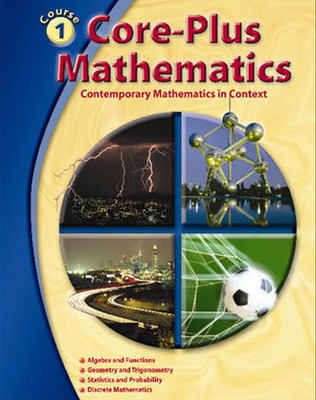Features:

• Development of mathematics as an active science of patterns involving quantity and change, shape and motion, data and chance, and counting and algorithms
• Courses organized around interwoven strands of
• algebra and functions
• geometry and trigonometry
• statistics and probability
• discrete mathematics
• Mathematical strands developed in coherent, focused units connected by fundamental unifying ideas
• Mathematics developed in context with an emphasis on applications and mathematical modeling
• Student-centered investigations promote active learning through problem solving
• Full and appropriate use of technology tools, including new CPMP-Tools® software for each course

## Program Components

•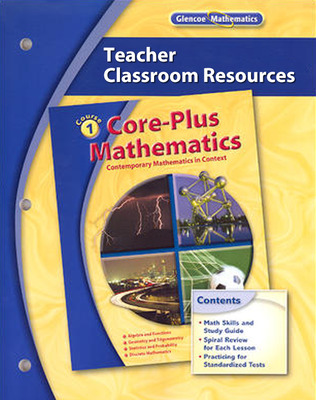Core-Plus Mathematics: Contemporary Mathematics In Context, Course 1, Teacher Classroom Resources
•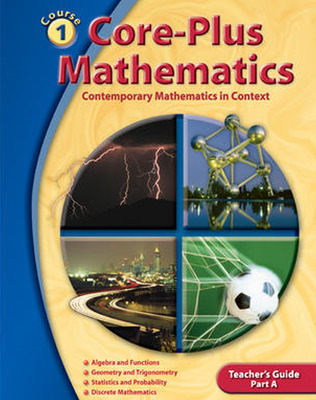Core-Plus Mathematics: Contemporary Mathematics In Context, Course 1 Part A, Teacher's Guide'
•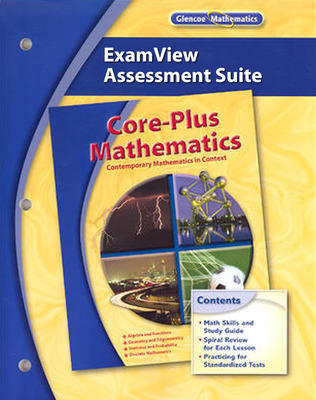Core-Plus Mathematics: Contemporary Mathematics in Context, Course 1-3 ExamView Assessment Suite CD-ROM
•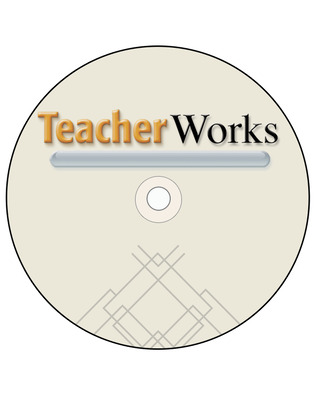Core-Plus Mathematics: Contemporary Mathematics in Context, Courses 1-3, TeacherWorks Plus CD-ROM
•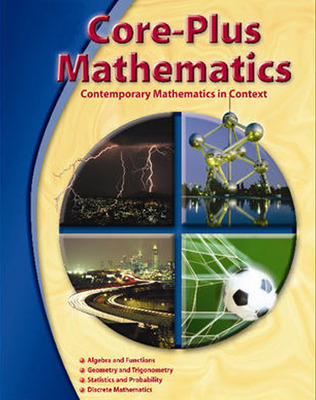Implementing the Core-Plus Mathematics Curriculum
•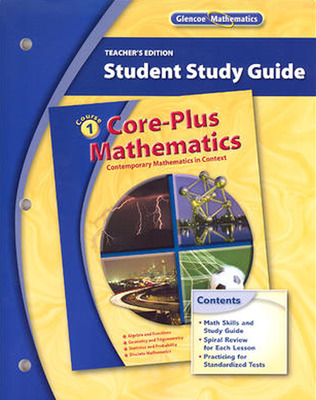CORE-PLUS MATHEMATICS COURSE 1 STUDENT STUDY GUIDE Teacher's EDITION'
•Core-Plus Mathematics Course 1, Student Edition
•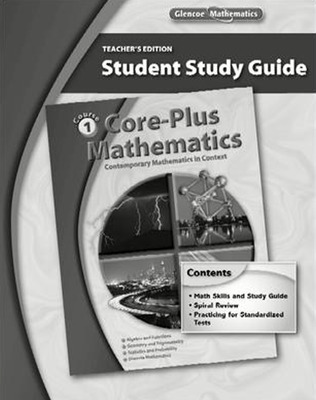Core-Plus Mathematics: Contemporary Mathematics In Context, Course 1, Student Study Guide
•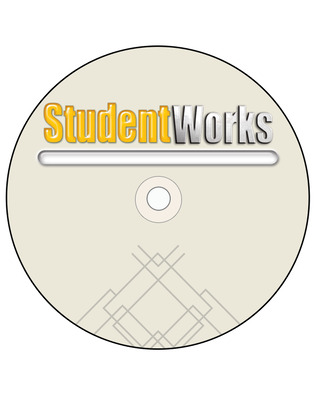Core-Plus Mathematics: Contemporary Mathematics in Context, Courses 1-3, StudentWorks CD-ROM
Sort by: